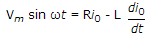# Electronics and Communication Engineering - Power Electronics

### Exercise :: Power Electronics - Section 2

41.

UJT can be used for

 A. firing an SCR B. turning off an SCR C. both firing and turning off an SCR D. none of the above

Explanation:

UJT relaxation oscillator is used to provide gate pulses to SCR for triggering.

42.

In a series inverter supplying a load resistance R, the commutating elements L and C should be such that

 A.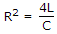B.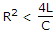C.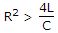D.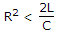Explanation:

Load should be under damped.

43.

In a full wave converter (M - 2 connection) feeding an RLE load, the peak thyristor current is 15 A. For fully controlled bridge circuit and same load current, the peak thyristor current will be

 A. 30 A B. 152 A C. 15 A D.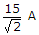Explanation:

In both the connections the output voltage and peak thyristor current are the same. Both are full wave rectifiers.

44.

Induction heating systems require low frequency inverters.

 A. True B. False

Explanation:

It uses high frequency inverter.

45.

A single phase half wave controlled rectifier circuit is feeding on RL load. The circuit has a freewheeling diode. The input voltage is v = Vm sin ωt. If I0 is the load current during the conduction of freewheeling diode, the KVL equation during the conduction of free wheeling diode is

 A.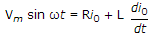B.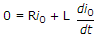C.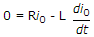D.